A-level Sample Test
Paper 2
How would you like to complete the test?
Check question by question
Check the test at the end
marks

State the values of |x| for which the binomial expansion of (3+2x)^{−4} is valid.

|x| < \frac{2}{3}
|x| < 1
|x| < \frac{3}{2}
|x| < 3
[1 mark] ?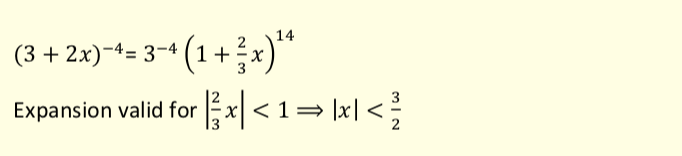marks

A zoologist is investigating the growth of a population of red squirrels in a forest.

She uses the equation N=\frac{200}{1+9e^{-\frac{t}{5}}} as a model to predict the number of squirrels, N, in the population t weeks after the start of the investigation.

What is the size of the squirrel population at the start of the investigation?

5
20
40
200
[1 mark] ?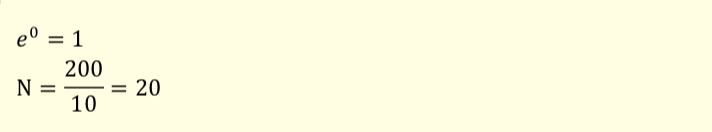marks

A curve is defined by the parametric equations

x = t^3 + 2 ,   y = t^2 − 1

(a)

Find the gradient of the curve at the point where t = −2

[4 marks] ?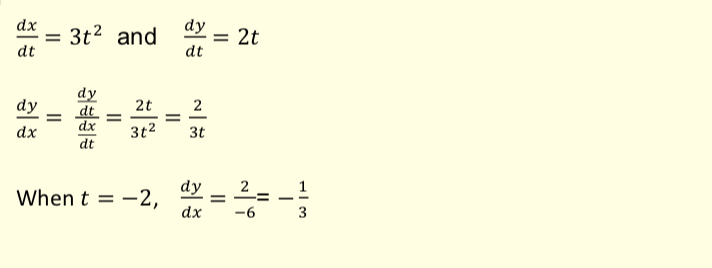(b)

Find a Cartesian equation of the curve.

[2 marks] ?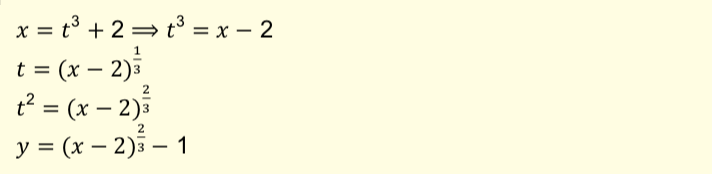marks

The equation x^3 − 3x + 1 = 0 has three real roots.

(a)

Calculate f(-2) and f(-1) and decide whether there is a root, x, such that −2 < x < −1

f(-2) =

f(-1) =

[2 marks] ?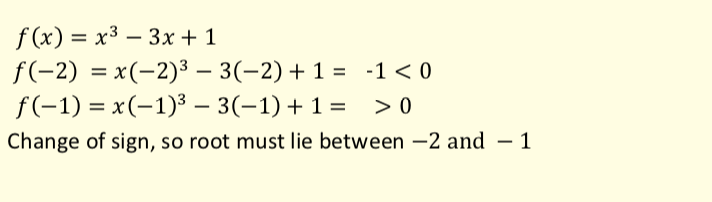(b)

Taking x_1 = −2 as the first approximation to one of the roots, use the Newton-Raphson method to find x_2, the second approximation.

[3 marks] ?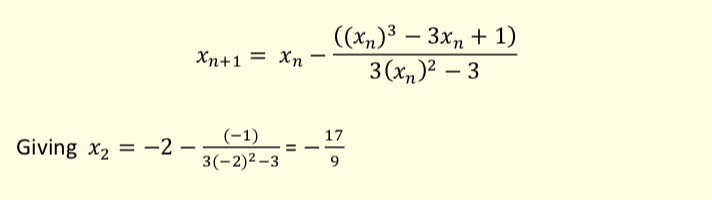(c)

Will this method work when the first approximation is x_1 = −1?

[1 mark] ?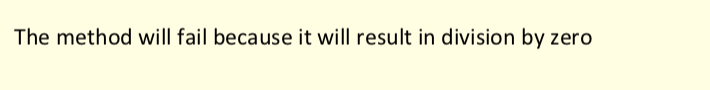marks
(a)

Express y = 3cos\theta + 3sin\theta in the form y = R cos(\theta − \alpha) where R > 0 and -\frac{\pi}{2}\le\alpha\le\frac{\pi}{2}

R =

𝛼 = [6 marks] ?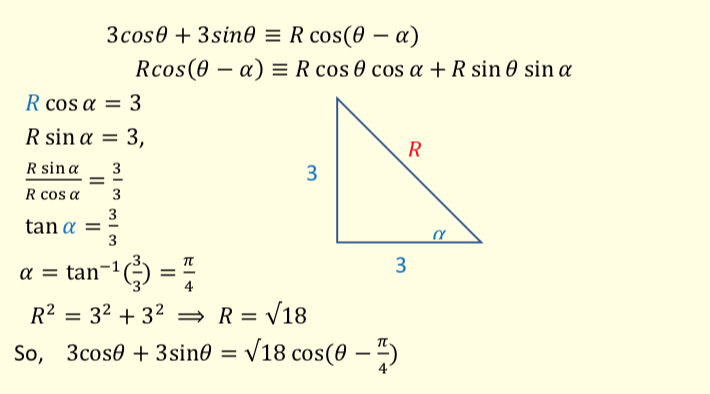(b)

Hence or otherwise find the least value and greatest value of 4 + (3cos\theta + 3sin\theta)^2

least value =

greatest value = [3 marks] ?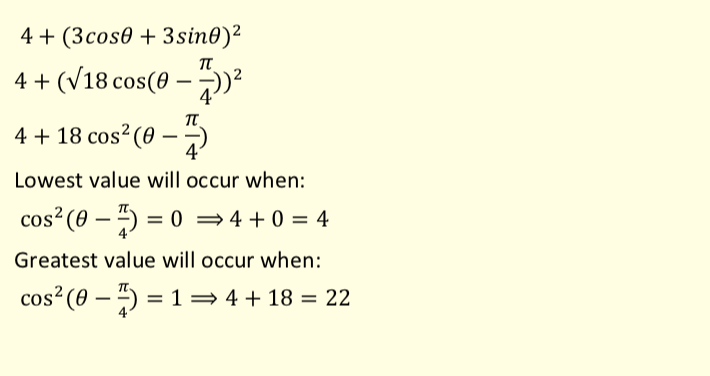marks

A curve C, has equation y = x^2 − 4x + k , where k is a constant.

It crosses the x-axis at the points (2 + \sqrt{5}, 0) and (2 − \sqrt{5}, 0)

Find the value of k.

k = [2 marks] ?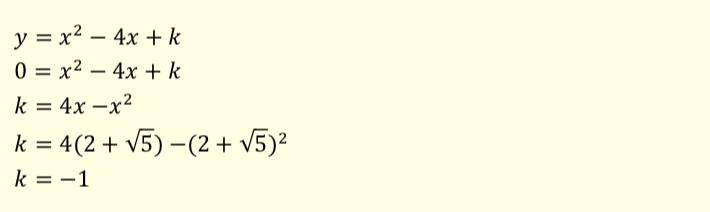marks

Using 2n + 1 and 2n + 3 (where n is a positve integer) as consecutive odd numbers, show that

(2n+1)+(2n+3)

can be expressed in the form

a^2 − b^2

where a and b are positive integers.

Give a and b in terms of n.

a =

b = [3 marks] ?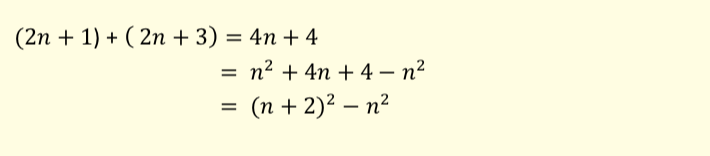marks

A curve has equation y=2x\cos3x+\left(3x^2−4\right)\sin3x

(a)
Find \frac{dy}{dx}, giving your answer in the form (mx^2 + n)\cos3x, where m and n are integers.

m =

n = [4 marks] ?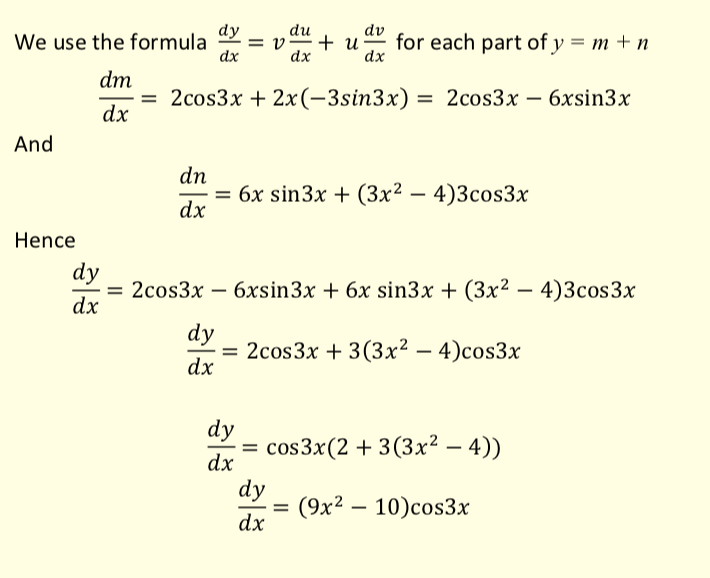(b)

Show that the x-coordinates of the points of inflection of the curve satisfy the equation

\cot ax = \frac{bx^2 − c}{6x}

Find a, b and c where a, b, and c are integers.

a =

b =

c = [4 marks] ?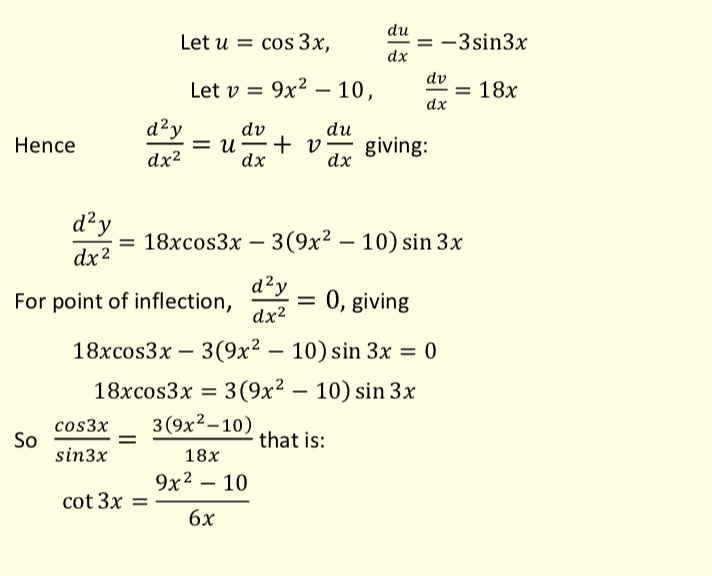marks

Three consecutive terms in an arithmetic sequence are 3e^{-p}, 5, 3e^p

Find the possible values of p. Give your answers in an exact form.

p = , [6 marks] ?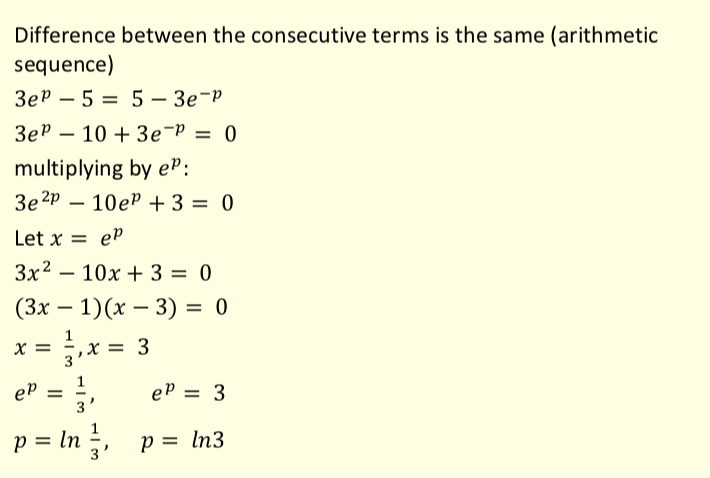marks

A single force of magnitude 4 newtons acts on a particle of mass 50 grams.

Find the magnitude of the acceleration of the particle.

12.5 ms−2
0.08 ms−2
0.0125 ms−2
80 ms−2
[1 mark] ?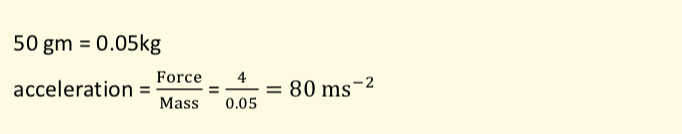marks

A uniform rod, AB, has length 3 metres and mass 24 kg.

A particle of mass M kg is attached to the rod at A.

The rod is balanced in equilibrium on a support at C, which is 0.8 metres from A.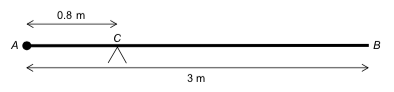Find the value of M.

M = [2 marks] ?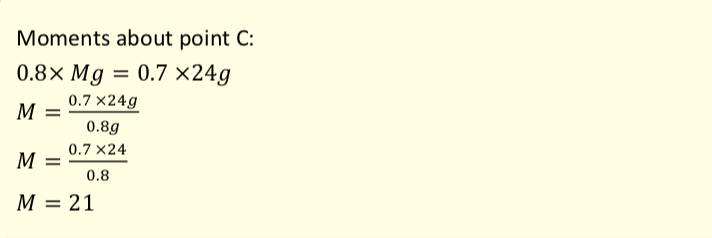marks

A particle moves on a straight line with a constant acceleration, a ms−2.

The initial velocity of the particle is U ms−1.

After T seconds the particle has velocity V ms−1.

This information is shown on the velocity-time graph.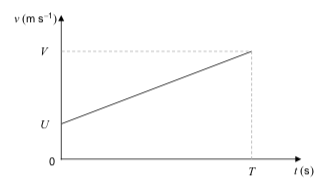The displacement, S metres, of the particle from its initial position at time T seconds is given by the formula

S=\frac{1}{2}(U+V)T

(a)

By considering the gradient of the graph, or otherwise, write down a formula for a in terms of U, V and T.

a = [1 mark] ?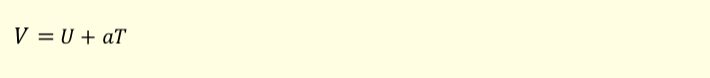(b)

Find a formula for V^2 in terms of

U, a and S

V^2 = [3 marks] ?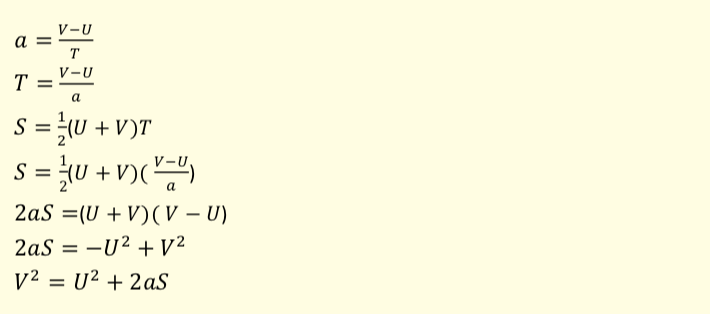marks

The three forces F1 , F2 and F3 are acting on a particle.

F1 = (25i + 12j)N

F2 = (–7i + 5j)N

F3 = (15i – 28j)N

The unit vectors i and j are horizontal and vertical respectively.

The resultant of these three forces is F newtons.

(a)
(i)

Find the magnitude of F, giving your answer to three significant figures.

N [2 marks] ?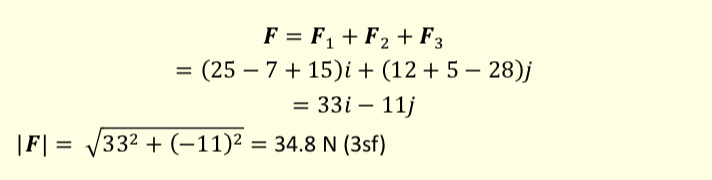(ii)

Find the acute angle that F makes with the horizontal, giving your answer to the nearest 0.1°

° [2 marks] ?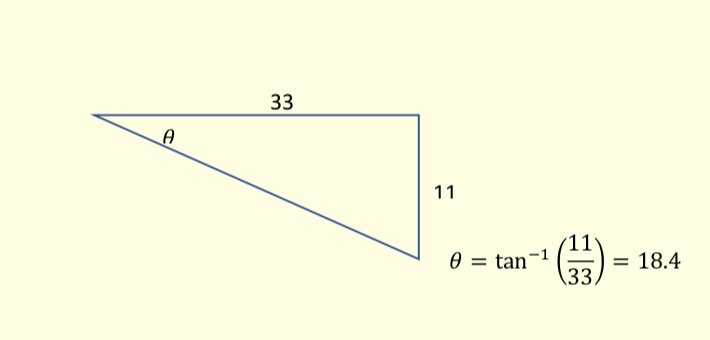(b)

The fourth force, F4, is applied to the particle so that the four forces are in equilibrium.

F4 = [1 mark] ?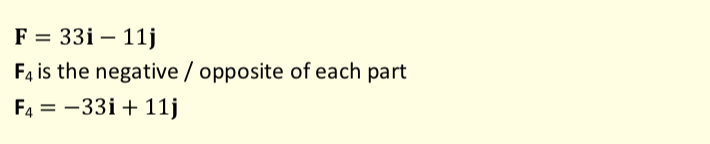marks

The graph below models the velocity of a small train as it moves on a straight track for 20 seconds.

The front of the train is at the point A when t = 0

The mass of the train is 800kg.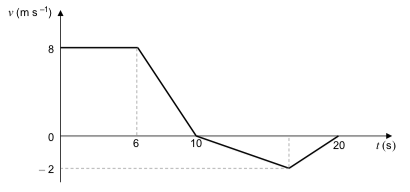(a)

Find the total distance travelled in the 20 seconds.

m [3 marks] ?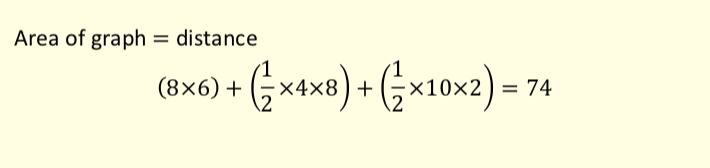(b)

Find the distance of the front of the train from the point A at the end of the 20 seconds.

m [1 mark] ?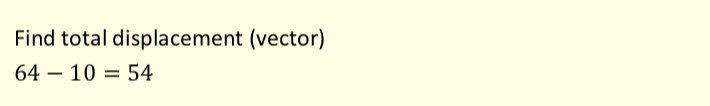(c)

Find the maximum magnitude of the resultant force acting on the train.

N [2 marks] ?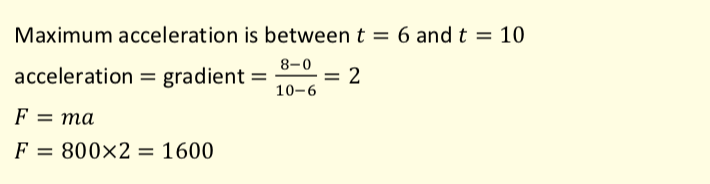marks

At time t = 0, a parachutist jumps out of an airplane that is travelling horizontally.

The velocity, v ms-1 , of the parachutist at time t seconds is given by:

\mathbf{v}=\left(40e^{-0.2t}\right)\mathbf{i}+50\left(e^{-0.2t}-1\right)\mathbf{j}

The unit vectors i and j are horizontal and vertical respectively.

Assume that the parachutist is at the origin when t = 0

Model the parachutist as a particle.

(a)

Find an expression for the position vector of the parachutist at time t.

r = i + j [4 marks] ?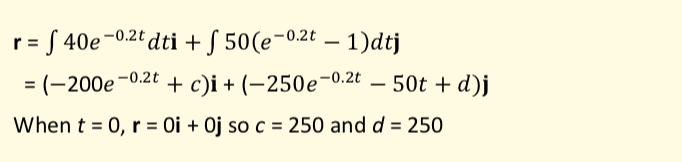(b)

The parachutist opens her parachute when she has travelled 100 metres horizontally.

Find the vertical displacement of the parachutist from the origin when she opens her parachute.

m [4 marks] ?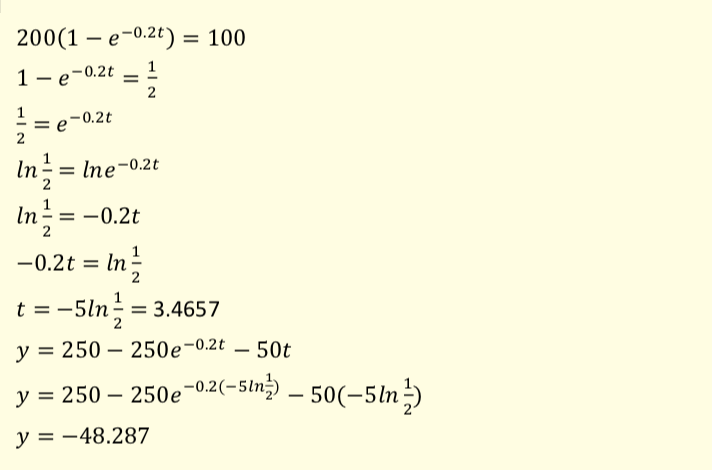(c)

Deduce the value of g used in the formulation of this model.

ms-2 [3 marks] ?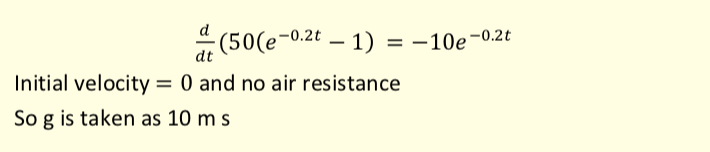marks

In this question use g = 9.8 ms−2.

The diagram shows a box, of mass 8.0 kg, being pulled by a string so that the box moves at a constant speed along a rough horizontal wooden board.

The string is at an angle of 40° to the horizontal.

The tension in the string is 50 newtons.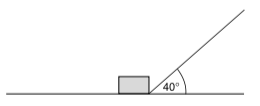The coefficient of friction between the box and the board is μ.

Model the box as a particle.

(a)

μ = [4 marks] ?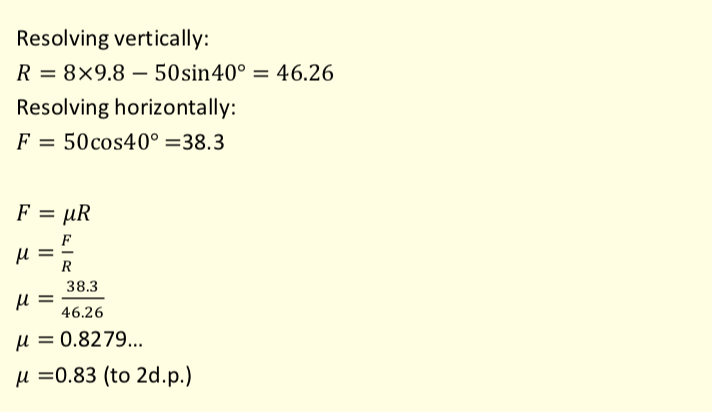(b)

One end of the board is lifted up so that the board is now inclined at an angle of 5° to the horizontal.

The box is pulled up the inclined board.

The string remains at an angle of 40° to the board.

The tension in the string is increased so that the box accelerates up the board at 3 ms-2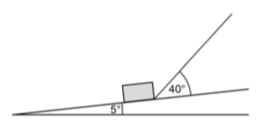Find the tension in the string as the box accelerates up the slope at 3 ms-2 giving your answer to 2 significant figures.

[7 marks] ?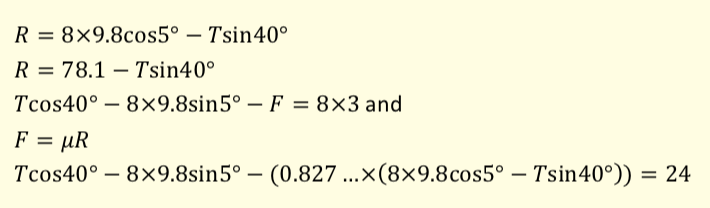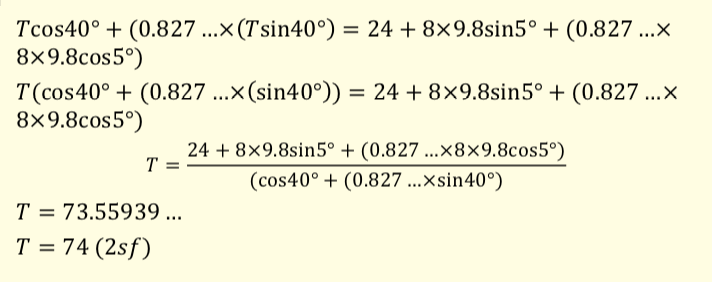marks

In this question use g = 9.81 ms−2.

A ball is projected from the origin. After 2.5 seconds, the ball lands at the point with position vector (40i − 10j) metres.

The unit vectors i and j are horizontal and vertical respectively.

Assume that there are no resistance forces acting on the ball.

(a)

Find the speed of the ball when it is at a height of 3 metres above its initial position giving your answer to 3 significant figures.

ms-1 [6 marks] ?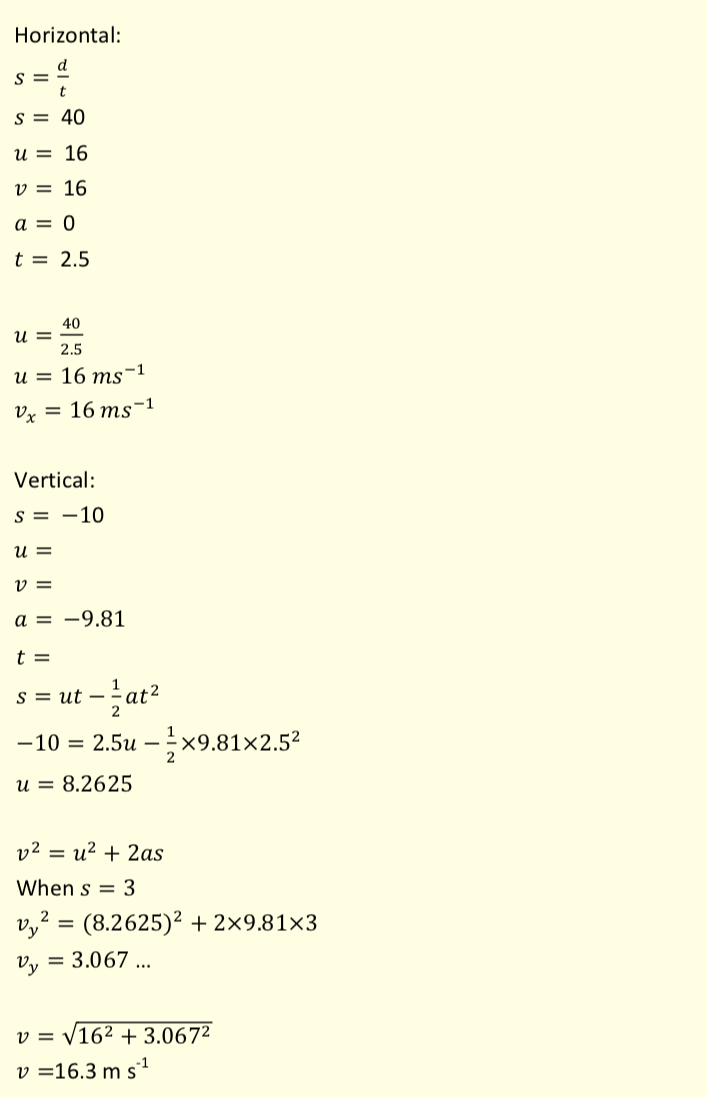(b)

State the speed of the ball when it is at its maximum height giving your answer to 2 significant figures.

ms-1 [1 mark] ?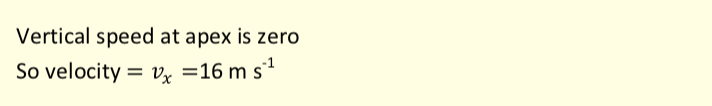Test check
Total: - of marks